The Australian Journal of Mathematical Analysis and Applications

 Home News Editors Volumes RGMIA Subscriptions Authors Contact

ISSN 1449-5910

You searched for jung
Total of 141 results found in site

39: Paper Source PDF document

Paper's Title:

Some Convergence Results for  Jungck-Am Iterative Process In Hyperbolic Spaces

Author(s):

Akindele Adebayo Mebawondu and Oluwatosin Temitope Mewomo

School of Mathematics, Statistics and Computer Science,
University of KwaZulu-Natal, Durban,
South Africa.
E-mail: 216028272@stu.ukzn.ac.za, mewomoo@ukzn.ac.za

Abstract:

In this paper, we introduce a new three steps iterative process called Jungck-AM iterative process and show that the proposed iterative process can be used to approximate fixed points of Jungck-contractive type mappings and Jungck-Suzuki type mappings. In addition, we establish some strong and Δ-convergence results for the approximation of fixed points of Jungck-Suzuki type mappings in the frame work of uniformly convex hyperbolic space. Furthermore, we show that the newly proposed iterative process has a better rate of convergence compare to the Jungck-Noor, Jungck-SP, Jungck-CR and some existing iterative processes in the literature. Finally, stability, data dependency results for Jungck-AM iterative process is established and we present an analytical proof and numerical examples to validate our claim.

11: Paper Source PDF document

Paper's Title:

Asymptotic Behavior of Mixed Type Functional Equations

Author(s):

J. M. Rassias

Pedagogical Department, E.E., National and Capodistrian University of Athens, Section of Mathematics And Informatics, 4, Agamemnonos Str., Aghia Paraskevi, Athens 15342,Greece
jrassias@primedu.uoa.gr
URL: http://www.primedu.uoa.gr/~jrassias/

Abstract:

In 1983 Skof  was the first author to solve the Ulam problem for additive mappings on a restricted domain. In 1998 Jung  investigated the Hyers-Ulam stability of additive and quadratic mappings on restricted domains. In this paper we improve the bounds and thus the results obtained by Jung , in 1998 and by the author , in 2002. Besides we establish new theorems about the Ulam stability of mixed type functional equations on restricted domains. Finally, we apply our recent results to the asymptotic behavior of functional equations of different types.

9: Paper Source PDF document

Paper's Title:

A Fixed Point Approach to the Stability of the Equation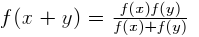Author(s):

Soon-Mo Jung

Mathematics Section, College of Science and Technology
Hong-Ik University, 339-701 Chochiwon
Republic of Korea.

smjung@hongik.ac.kr

Abstract:

We will apply a fixed point method for proving the Hyers--Ulam stability of the functional equation.

6: Paper Source PDF document

Paper's Title:

New Coincidence and Fixed Point Theorems for Strictly Contractive Hybrid Maps

Author(s):

S. L. Singh and Amal M. Hashim

21, Govind Nagar, Rishikesh 249201,
Ua, India
vedicmri@sancharnet.in

Dept. of Math., College of Science,
Univ. of Basarah,
Iraq.

Abstract:

The purpose of this paper is to study the (EA)-property and noncompatible maps of a hybrid pair of single-valued and multivalued maps in fixed point considerations. Such maps have the remarkable property that they need not be continuous at their common fixed points. We use this property to obtain some coincidence and fixed point theorems for strictly contractive hybrid maps without using their continuity and completeness or compactness of the space.

6: Paper Source PDF document

Paper's Title:

Ulam Stability of Functional Equations

Author(s):

Stefan Czerwik and Krzysztof Król

Institute of Mathematics
Silesian University of Technology
Kaszubska 23, 44-100 Gliwice,
Poland

Stefan.Czerwik@polsl.pl
Krzysztof.Krol@polsl.pl

Abstract:

In this survey paper we present some of the main results on Ulam-Hyers-Rassias stability for important functional equations.

6: Paper Source PDF document

Paper's Title:

Common Fixed Point Results for Banach Operator Pairs and Applications to Best Approximation

Author(s):

Hemant Kumar Nashine

Department of Mathematics,
Disha Institute of Management and Technology,
Satya Vihar, Vidhansabha - Chandrakhuri Marg (Baloda Bazar Road), Mandir Hasaud,
Raipur - 492101(Chhattisgarh), India.

hemantnashine@rediffmail.com
nashine_09@rediffmail.com

Abstract:

The common fixed point results for Banach operator pair with generalized nonexpansive mappings in q-normed space have been obtained in the present work. As application, some more general best approximation results have also been determined without the assumption of linearity or affinity of mappings. These results unify and generalize various existing known results with the aid of more general class of noncommuting mappings.

6: Paper Source PDF document

Paper's Title:

Ulam Stability of Reciprocal Difference and Adjoint Functional Equations

Author(s):

K. Ravi, J. M. Rassias and B. V. Senthil Kumar

Department of Mathematics,
Sacred Heart College, Tirupattur - 635601,
India

Pedagogical Department E. E.,
Section of Mathematics and Informatics,
National and Capodistrian University of Athens,
Athens, Attikis 15342,
GREECE

Department of Mathematics,
C.Abdul Hakeem College of Engineering and
Technology, Melvisharam - 632 509, India

shckavi@yahoo.co.in
jrassias@primedu.uoa.gr
bvssree@yahoo.co.in

Abstract:

In this paper, the reciprocal difference functional equation (or RDF equation) and the reciprocal adjoint functional equation (or RAF equation) are introduced. Then the pertinent Ulam stability problem for these functional equations is solved, together with the extended Ulam (or Rassias) stability problem and the generalized Ulam (or Ulam-Gavruta-Rassias) stability problem for the same equations.

5: Paper Source PDF document

Paper's Title:

Applications of Relations and Relators in the Extensions of Stability Theorems for Homogeneous and Additive Functions

Author(s):

Árpád Száz

Institute of Mathematics, University of Debrecen,
H-4010 Debrecen, Pf. 12,
Hungary
szaz@math.klte.hu

Abstract:

By working out an appropriate technique of relations and relators and extending the ideas of the direct methods of Z. Gajda and R. Ger, we prove some generalizations of the stability theorems of D. H. Hyers, T. Aoki, Th. M. Rassias and P. Găvruţă in terms of the existence and unicity of 2-homogeneous and additive approximate selections of generalized subadditive relations of semigroups to vector relator spaces. Thus, we obtain generalizations not only of the selection theorems of Z. Gajda and R. Ger, but also those of the present author.

5: Paper Source PDF document

Paper's Title:

A Generalization of a Trace Inequality for Positive Definite Matrices

Author(s):

E. V. Belmega, M. Jungers, and S. Lasaulce

Université Paris-Sud Xi, SUPELEC,
Laboratoire Des Signaux Et Systčmes,
Gif-Sur-Yvette,
France.

belmega@lss.supelec.fr
http://veronica.belmega.lss.supelec.fr

CNRS, ENSEM, CRAN, Vandoeuvre,
France.

marc.jungers@cran.uhp-nancy.fr
http://perso.ensem.inpl-nancy.fr/Marc.Jungers/

CNRS, SUPELEC, Laboratoire des Signaux et Systčmes,
Gif-Sur-Yvette,
France.

lasaulce@lss.supelec.fr
http://samson.lasaulce.lss.supelec.fr

Abstract:

In this note, we provide a generalization of the trace inequality derived in [Belmega].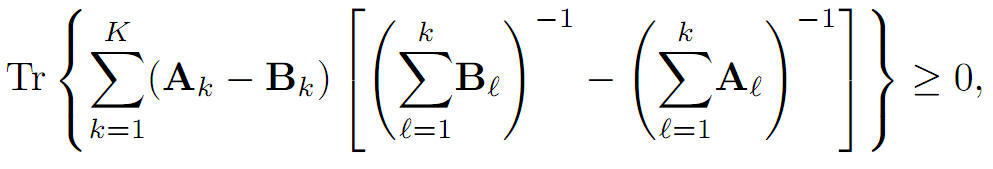More precisely, we prove that for arbitrary K ≥ 1 where Tr(∙) denotes the matrix trace operator, A1, B1 are any positive definite matrices and Ak, Bk for all k∈{2,...,k}, are any positive semidefinite matrices.

4: Paper Source PDF document

Paper's Title:

Komatu Integral Transforms of Analytic Functions Subordinate to Convex Functions

Author(s):

T. N. Shanmugam and C. Ramachandran

Department of Mathematics, College of Engineering,
India
shan@annauniv.edu

Department of Mathematics, College of Engineering,
India
crjsp2004@yahoo.com

Abstract:

In this paper, we consider the class A of the functions f(z) of the form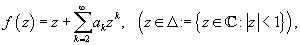which are analytic in an open disk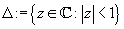and study certain subclass of the class A, for which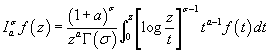has some property. Certain inclusion and the closure properties like convolution with convex univalent function etc. are studied.

4: Paper Source PDF document

Paper's Title:

On the Generalized Stability and Asymptotic Behavior of Quadratic Mappings

Author(s):

Hark-Mahn Kim, Sang-Baek Lee and Eunyoung Son

Department of Mathematics
Chungnam National University
Daejeon, 305-764,
Republic of Korea

hmkim@cnu.ac.kr

Abstract:

We extend the stability of quadratic mappings to the stability of general quadratic mappings with several variables, and then obtain an improved asymptotic property of quadratic mappings on restricted domains.

4: Paper Source PDF document

Paper's Title:

Hyers-Ulam-Rassias Stability of a Generalized Jensen Functional Equation

Author(s):

A. Charifi, B. Bouikhalene, E. Elqorachi and A. Redouani

Department of
Mathematics, Faculty of Sciences,
Ibn Tofail University,
Kenitra, Morocco
charifi2000@yahoo.fr bbouikhalene@yahoo.fr

Department of
Mathematics, Faculty of Sciences,
Ibn Zohr University,
elqorachi@hotmail.com Redouani-ahmed@yahoo.fr

Abstract:

In this paper we obtain the Hyers-Ulam-Rassias stability for the generalized Jensen's functional equation in abelian group (G,+). Furthermore we discuss the case where G is amenable and we give a note on the Hyers-Ulam-stability of the K-spherical (n × n)-matrix functional equation.

4: Paper Source PDF document

Paper's Title:

Approximation of an AQCQ-Functional Equation and its Applications

Author(s):

Choonkil Park and Jung Rye Lee

Department of Mathematics,
Research Institute for Natural Sciences,
Hanyang University, Seoul 133-791,
Korea;

Department of Mathematics,
Daejin University,
Kyeonggi 487-711,
Korea

baak@hanyang.ac.kr
jrlee@daejin.ac.kr

Abstract:

This paper is a survey on the generalized Hyers-Ulam stability of an AQCQ-functional equation in several spaces. Its content is divided into the following sections:

1. Introduction and preliminaries.

2. Generalized Hyers-Ulam stability of an AQCQ-functional equation in Banach spaces: direct method.

3. Generalized Hyers-Ulam stability of an AQCQ-functional equation in Banach spaces: fixed point method.

4. Generalized Hyers-Ulam stability of an AQCQ-functional equation in random Banach spaces: direct method.

5. Generalized Hyers-Ulam stability of an AQCQ-functional equation in random Banach spaces: fixed point method.

6. Generalized Hyers-Ulam stability of an AQCQ-functional equation in non-Archi-medean Banach spaces: direct method.

7. Generalized Hyers-Ulam stability of an AQCQ-functional equation in non-Archi-medean Banach spaces: fixed point method.

4: Paper Source PDF document

Paper's Title:

Characterization of Caristi Type Mapping Through its Absolute Derivative

Author(s):

M. Muslikh1, A. Kilicman2,3, S. H. Sapar4 and N. Bacho5

1Department of Mathematics,
University of Brawijaya,
Malang 65143, East Java,
Indonesia.
E-mail: mslk@ub.ac.id

2Department of Mathematics,
Universiti Putra Malaysia,
43400 UPM, Serdang, Selangor,
Malaysia
E-mail: akilic@upm.edu.my

3Department of Electrical and Electronic Engineering,
Istanbul Gelisim University,
Avcilar, Istanbul,
Turkey

4Department of Mathematics,
Universiti Putra Malaysia,
43400 UPM, Serdang, Selangor,
Malaysia
E-mail: sitihas@upm.edu.my

5Department of Mathematics,
Universiti Putra Malaysia,
43400 UPM, Serdang, Selangor,
Malaysia
E-mail: norfifah@upm.edu.my

Abstract:

The purpose of this article to characterize the Caristi type mapping by the absolute derivative. The equivalences of the Caristi mapping with contraction mapping is discussed too. In addition, it was shown that the contraction mapping can be tested through its absolute derivative.

4: Paper Source PDF document

Paper's Title:

Pointwise Convergence of Fourier-type Series with Exponential Weights

Author(s):

Hee Sun Jung and Ryozi Sakai

Department of Mathematics Education, Sungkyunkwan University,
Seoul 110-745,
Republic of Korea.
E-mail: hsun90@skku.edu

Department of Mathematics,
Meijo University, Nagoya 468-8502,
Japan.
E-mail: ryozi@hm.aitai.ne.jp

Abstract:

Let R = ( - ∞,∞), and let Q∈C1(R):R→[0,∞) be an even function. We consider the exponential weights w(x)=e-Q(x), xR. In this paper we obtain a pointwise convergence theorem for the Fourier-type series with respect to the orthonormal polynomials {pn(w2;x)}.

3: Paper Source PDF document

Paper's Title:

Fixed Point Theorems for a Finite Family of Asymptotically Nonexpansive Mappings

Author(s):

E. Prempeh

Department of Mathematics,
Kwame Nkrumah University of Science and Technology,
Kumasi, Ghana
edward_prempeh2000@yahoo.com

Abstract:

Let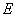be a real reflexive Banach space with a uniformly Gâteaux differentiable norm,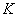be a nonempty bounded closed convex subset of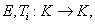i=1,2,...,r be a finite family of asymptotically nonexpansive mappings such that for each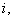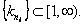Let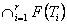be a nonempty set of common fixed points of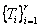and define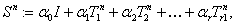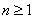. Let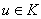be fixed and let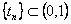be such that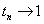as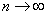. We can prove that the sequence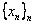satisfying the relation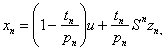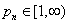associated with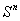, converges strongly to a fixed point of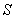providedpossesses uniform normal structure. Furthermore we prove that the iterative process: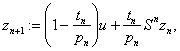, converges strongly to a fixed point of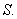3: Paper Source PDF document

Paper's Title:

A general common fixed point theorem for reciprocally continuous mappings satisfying an implicit relation

Author(s):

A. Djoudi and A. Aliouche

Faculty of Science, University of Annaba,
P.O. Box 23000, Annaba,
Algeria.

Department of Mathematics, University of Larbi Ben M'Hidi,
Oum-El-Bouaghi 04000,
Algeria.
abdmath@hotmail.com

Abstract:

A general common fixed point theorem for compatible mappings satisfying an implicit relation is obtained by replacing the continuity of one mapping by the reciprocal continuity of two mappings.

2: Paper Source PDF document

Paper's Title:

On the Ulam Stability for Euler-Lagrange Type Quadratic Functional Equations

Author(s):

Matina John Rassias and John Michael Rassias

Statistics and Modelling Science,
University of Strathclyde,
Livingstone Tower,
26 Richmond Str,
Glasgow, Uk, G1 1xh

Pedagogical Department, E. E., National and Capodistrian University of Athens,
Section of Mathematics and Informatics,
Athens 15342, Greece

Abstract:

In 1940 (and 1968) S. M. Ulam proposed the well-known Ulam stability problem. In 1941 D.H. Hyers solved the Hyers-Ulam problem for linear mappings. In 1951 D. G. Bourgin has been the second author treating the Ulam problem for additive mappings. In 1978 according to P.M. Gruber this kind of stability problems is of particular interest in probability theory and in the case of functional equations of different types. In 1982-2004 we established the Hyers-Ulam stability for the Ulam problem for different mappings. In 1992-2000 J.M. Rassias investigated the Ulam stability for Euler-Lagrange mappings. In this article we solve the Ulam problem for Euler-Lagrange type quadratic functional equations. These stability results can be applied in mathematical statistics, stochastic analysis, algebra, geometry, as well as in psychology and sociology.

2: Paper Source PDF document

Paper's Title:

Solution of the Hyers-Ulam Stability Problem for Quadratic Type Functional Equations in Several Variables

Author(s):

John Michael Rassias

Pedagogical Department, E.E., National and Capodistrian University of Athens,
Section of Mathematics and Informatics,
Athens 15342,
Greece jrassias@primedu.uoa.gr
URL: http://www.primedu.uoa.gr/~jrassias/

Abstract:

In 1940 (and 1968) S. M. Ulam proposed the well-known Ulam stability problem. In 1941 D. H. Hyers solved the Hyers-Ulam problem for linear mappings. In 1951 D. G. Bourgin has been the second author treating the Ulam problem for additive mappings. In 1978 according to P. M. Gruber this kind of stability problems is of particular interest in probability theory and in the case of functional equations of different types. In 1982-2004 we established the Hyers-Ulam stability for the Ulam problem for different mappings. In this article we solve the Hyers-Ulam problem for quadratic type functional equations in several variables. These stability results can be applied in stochastic analysis, financial and actuarial mathematics, as well as in psychology and sociology.

2: Paper Source PDF document

Paper's Title:

A Stability of the G-type Functional Equation

Author(s):

Gwang Hui Kim

Department of Mathematics, Kangnam University
Suwon 449-702, Korea.
ghkim@kangnam.ac.kr

Abstract:

We will investigate the stability in the sense of Găvruţă for the G-type functional equation f(φ(x))=Γ(x)f(x)+ψ(x) and the stability in the sense of Ger for the functional equation of the form f(φ(x))=Γ(x)f(x). As a consequence, we obtain a stability results for G-function equation.

2: Paper Source PDF document

Paper's Title:

Iterative Approximation of Zeros of Accretive Type Maps, with Applications

Author(s):

Charles Ejike Chidume, Chinedu Godwin Ezea, and Emmanuel Ezzaka Otubo

African University of Science and Technology, Abuja,
Nigeria.
E-mail: cchidume@aust.edu.ng
E-mail: chinedu.ezea@gmail.com
E-mail: mrzzaka@yahoo.com

Department of Mathematics,
Nnamdi Azikiwe University,
Awka,
Nigeria
E-mail: chinedu.ezea@gmail.com

Ebonyi State University,
Abakaliki,
Nigeria
E-mail: mrzzaka@yahoo.com

Abstract:

Let E be a reflexive real Banach space with uniformly Gâteaux differentiable norm. Let J:E E* be the normalized duality map on E and let A:E* E be a map such that AJ is an accretive and uniformly continuous map. Suppose that (AJ)-1(0) in nonempty. Then, an iterative sequence is constructed and proved to converge strongly to some u* in (AJ)-1(0). Application of our theorem in the case that E is a real Hilbert space yields a sequence which converges strongly to a zero of A. Finally, non-trivial examples of maps A for which AJ is accretive are presented..

2: Paper Source PDF document

Paper's Title:

Iterative Algorithm for Split Generalized Mixed Equilibrium Problem Involving Relaxed Monotone Mappings in Real Hilbert Spaces

Author(s):

1U.A. Osisiogu, F.L. Adum, and 2C. Izuchukwu

1Department of Mathematics and Computer Science,
Ebonyi State University, Abakaliki,
Nigeria.

2School of Mathematics, Statistics and Computer Science,
University of KwaZulu-Natal, Durban,
South Africa.
E-mail: izuchukwuc@ukzn.ac.za, izuchukwu_c@yahoo.com

Abstract:

The main purpose of this paper is to introduce a certain class of split generalized mixed equilibrium problem involving relaxed monotone mappings. To solve our proposed problem, we introduce an iterative algorithm and obtain its strong convergence to a solution of the split generalized mixed equilibrium problems in Hilbert spaces. As special cases of the proposed problem, we studied the proximal split feasibility problem and variational inclusion problem.

2: Paper Source PDF document

Paper's Title:

Existence of Solution of Differential and Riemann-Liouville Equation Via Fixed Point Approach in Complex Valued b-Metric Spaces

Author(s):

K. Afassinou, A. A. Mebawondu, H. A. Abass and O. K. Narain

Department of Science Access,
South Africa.
E-mail: komia@aims.ac.za

DST-NRF Centre of Excellence in Mathematical and Statistical Sciences (CoE-MaSS),
Johannesburg,
South Africa.
E-mail: dele@aims.ac.za

DST-NRF Centre of Excellence in Mathematical and Statistical Sciences (CoE-MaSS),
Johannesburg,
South Africa.
E-mail: hammedabass548@gmail.com

School of Mathematics, Statistics and Computer Science,
University of KwaZulu-Natal, Durban,
South Africa.
E-mail: naraino@ukzn.ac.za

Abstract:

In this paper, we establish some fixed point and common fixed point results for a new type of generalized contractive mapping using the notion of C-class function in the framework of complex valued b-metric spaces. As an application, we establish the existence and uniqueness of a solution for Riemann-Liouville integral and ordinary differential equation in the framework of a complete complex valued b-metric spaces. The obtained results generalize and improve some fixed point results in the literature.

1: Paper Source PDF document

Paper's Title:

On Stan Ulam and his Mathematics

Author(s):

Krzysztof Ciesielski and Themistocles M. Rassias

Mathematics Institute, Jagiellonian University,
Ł
jasiewicza 6, 30-348 Kraków,
Poland
Department of Mathematics. National Technical University of Athens,
Zografou Campus, 15780 Athens,
Greece

Krzysztof.Ciesielski@im.uj.edu.pl
trassias@math.ntua.gr

Abstract:

In this note we give a glimpse of the curriculum vitae of Stan Ulam, his personality and some of the mathematics he was involved in.

1: Paper Source PDF document

Paper's Title:

On a Method of Proving the Hyers-Ulam Stability of Functional Equations on Restricted Domains

Author(s):

Janusz Brzdęk

Department of Mathematics
Pedagogical University Podchor
ąźych 2,
30-084 Krak
ów,
Poland
jbrzdek@ap.krakow.pl

Abstract:

We show that generalizations of some (classical) results on the Hyers-Ulam stability of functional equations, in several variables, can be very easily derived from a simple result on stability of a functional equation in single variable

1: Paper Source PDF document

Paper's Title:

Fixed Points and Stability of the Cauchy Functional Equation

Author(s):

Choonkil Park and Themistocles M. Rassias

Department of Mathematics, Hanyang University,
Seoul 133-791,
Republic of Korea

Department of Mathematics, National Technical University of Athens,
Zografou Campus, 15780 Athens,
Greece

baak@hanyang.ac.kr
trassias@math.ntua.gr

Abstract:

Using fixed point methods, we prove the generalized Hyers-Ulam stability of homomorphisms in Banach algebras and of derivations on Banach algebras for the Cauchy functional equation.

1: Paper Source PDF document

Paper's Title:

An Efficient Modification of Differential Transform Method for Solving Integral and Integro-differential Equations

Author(s):

S. Al-Ahmad, Ibrahim Mohammed Sulaiman*, and M. Mamat

Faculty of Informatics and Computing,
Universiti Sultan Zainal Abidin,
Terengganu, Besut Campus, 22200,
Malaysia.

Abstract:

In this paper, classes of integral and integro-differential equations are solved using a modified differential transform method. This proposed technique is based on differential transform method (DTM), Laplace transform (LT) procedure and Pad\'{e} approximants (PA). The proposed method which gives a good approximation for the true solution in a large region is referred to modified differential transform method (MDTM). An algorithm was developed to illustrate the flow of the proposed method. Some numerical problems are presented to check the applicability of the proposed scheme and the obtained results from the computations are compared with other existing methods to illustrates its efficiency. Numerical results have shown that the proposed MDTM method is promising compared to other existing methods for solving integral and integro-differential equations.

1: Paper Source PDF document

Paper's Title:

Author(s):

F. U. Ogbuisi

School of Mathematics, Statistics and Computer Science,
University of KwaZulu-Natal, Durban,
South Africa.

Department of Mathematics,
University of Nigeria, Nsukka,
Nigeria.
E-mail: ferdinard.ogbuisi@unn.edu.ng fudochukwu@yahoo.com

Abstract:

In this paper, we propose and analyze a type of subgradient extragradient algorithm for the approximation of a solution of variational inequality problem which is also a common fixed point of an infinite family of relatively nonexpansive mappings in 2-uniformly convex Banach spaces which are uniformly smooth. By using the generalized projection operator, we prove a strong convergence theorem which does not require the prior knowledge of the Lipschitz constant of cost operator. We further applied our result to constrained convex minimization problem, convex feasibility problem and infinite family of equilibrium problems. Our results improve and complement related results in 2-uniformly convex and uniformly smooth Banach spaces and Hilbert spaces.

1: Paper Source PDF document

Paper's Title:

ψ(m,q)-Isometric Mappings on Metric Spaces

Author(s):

Sid Ahmed Ould Beinane, Sidi Hamidou Jah and Sid Ahmed Ould Ahmed Mahmoud

Mathematical Analysis and Applications, Mathematics Department, College of Science,
Jouf University,
Sakaka P.O.Box 2014,
Saudi Arabia.
E-mail: beinane06@gmail.com

Department of Mathematics, College of Science Qassim University,
P.O. Box 6640, Buraydah 51452,
Saudi Arabia.
E-mail: jahsiidi@yahoo.fr

Mathematical Analysis and Applications,
Mathematics Department, College of Science, Jouf University,
Sakaka P.O.Box 2014,
Saudi Arabia.
E-mail: sidahmed@ju.edu.sa, sidahmed.sidha@gmail.com

Abstract:

The concept of (m,p)-isometric operators on Banach space was extended to (m,q)-isometric mappings on general metric spaces in . This paper is devoted to define the concept of ψ(m, q)-isometric, which is the extension of A(m, p)-isometric operators on Banach spaces introduced in . Let T,ψ: (E,d) -> (E, d) be two mappings.
For some positive integer m and q (0,). T is said to be an ψ(m,q)-isometry, if for all  y,z E,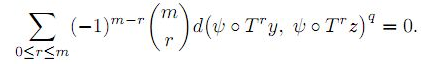Search and serve lasted 1 second(s).